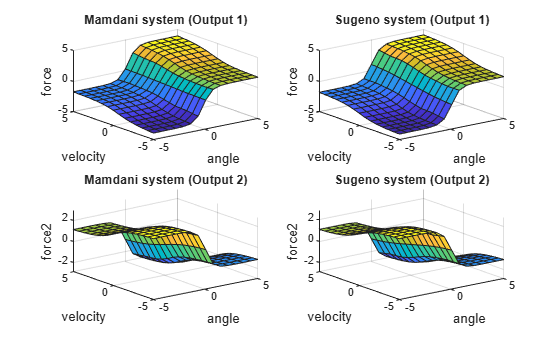Documentation

# convertToSugeno

Transform Mamdani fuzzy inference system into Sugeno fuzzy inference system

## Syntax

``sugenoFIS = convertToSugeno(mamdaniFIS)``

## Description

example

````sugenoFIS = convertToSugeno(mamdaniFIS)` transforms a Mamdani fuzzy inference system `mamdaniFIS` into a Sugeno fuzzy inference system `sugenoFIS`.```

## Examples

collapse all

Load a Mamdani fuzzy inference system.

`mam_fismat = readfis('mam22.fis');`

Convert this system to a Sugeno fuzzy inference system.

`sug_fismat = convertToSugeno(mam_fismat);`

Plot the output surfaces for both fuzzy systems.

```subplot(2,2,1) gensurf(mam_fismat) title('Mamdani system (Output 1)') subplot(2,2,2) gensurf(sug_fismat) title('Sugeno system (Output 1)') subplot(2,2,3) gensurf(mam_fismat,gensurfOptions('OutputIndex',2)) title('Mamdani system (Output 2)') subplot(2,2,4) gensurf(sug_fismat,gensurfOptions('OutputIndex',2)) title('Sugeno system (Output 2)')```The output surfaces for both systems are similar.

## Input Arguments

collapse all

Mamdani fuzzy inference system, specified as a `mamfis` object. Construct `mamdaniFIS` at the command line or using Fuzzy Logic Designer. For more information, see Build Fuzzy Systems at the Command Line and Build Fuzzy Systems Using Fuzzy Logic Designer.

## Output Arguments

collapse all

Sugeno fuzzy inference system, returned as a `sugfis` object. `sugenoFIS`:

• Has constant output membership functions, whose values correspond to the centroids of the output membership functions in `mamdaniFIS`

• Uses the weighted-average defuzzification method

• Uses the product implication method

• Uses the sum aggregation method

The remaining properties of `sugenoFIS`, including the input membership functions and rule definitions remain unchanged from `mamdaniFIS`.

## Tips

• If you have a functioning Mamdani fuzzy inference system, consider using `convertToSugeno` to convert to a more computationally efficient Sugeno structure to improve performance.

• If `sugFIS` has a single output variable and you have appropriate measured input/output training data, you can tune the membership function parameters of `sugFIS` using `anfis`.

Download now